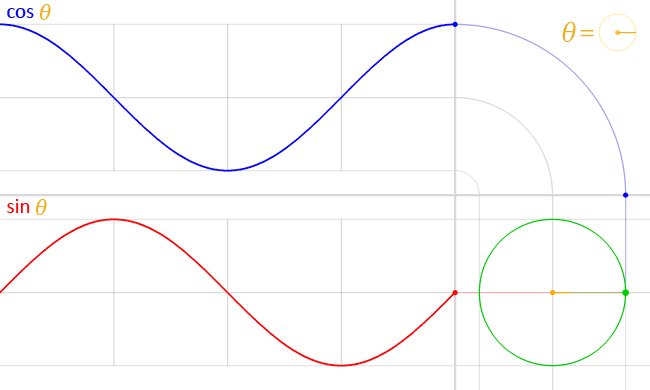# Smooth nature snake – game re-dev with Kotlin (part 2)

S

This is the second part of creating a smooth nature snake game. You can read the first part here. In this part, we will cover the challenges in simulating the snake’s movement pattern.

### How snakes move

Snakes move in zigzag patterns as demonstrated in the following figure.

We can see that the whole body of the snake forms a shape that is similar to the sine graph.

The sine function is more suitable for serpentine movement but not quite for side-winding. If you do care about the latter, I have tried a couple of equations to get it done and will cover that in another article.

Now we go for the serpentine movement pattern. “How to simulate such movement in code?”

From the animation, it is not hard to see that the body follows its head. When the snake moves, the head moves forward and followed by other body parts. Similarly, we can first calculate the head’s next position and then the other body path can follow the previous location of the head.

Here is the pseudo-code:

OK then, to calculate the position of the head in the real game, we will need more than just a sine function. But to make it simple, let ‘s go for the easiest case first: calculate position supposed that the snake is moving horizontally.

The following equation is to draw the sinusoidal graph based on the phase.

$$y = A sin(p_t)$$

Where $latexA\) is the amplitude,$latexp_t\) is the phase at time $latext\), and$latexy\) is the amplitude at the time $latext\)Draw sine and cosine graph based on the phase (angle) To implement this, we will define two variables, i.e. amplitude and phase. To update the phase, we increase “mHeadRadial” by a fixed radial value every step. The current amplitude (snake ‘s y coordinate) is dyOrigin. ### Calculate the actual position of the head Above we described how to calculate the current amplitude of the head in case of the horizontal movement. In the game, the snake can go to any direction while keeping the movement pattern. Therefore, we need to calculate the actual point of the head based on the movement direction. Namely, how can we translate the current (dxOrigin, dyOrigin) to the new coordinates? The angle between the movement vector and the$latexx\) axis is \$latex\theta\).

Thank mathematics, we can achieve that by using the axis rotation equations.

$$x’ = x \cos \theta + y \sin \theta$$

$$y’ = – x \sin \theta + y \cos \theta$$

The code for rotating axes is as follows:

And the code to update the head position based on movement vector.

### To be continued…

So in this article, we have covered the smooth nature snake’s movement by using the head-first approach. Another tricky part is to draw the snake’s head that is turning to the movement direction. We will cover this in part 3.